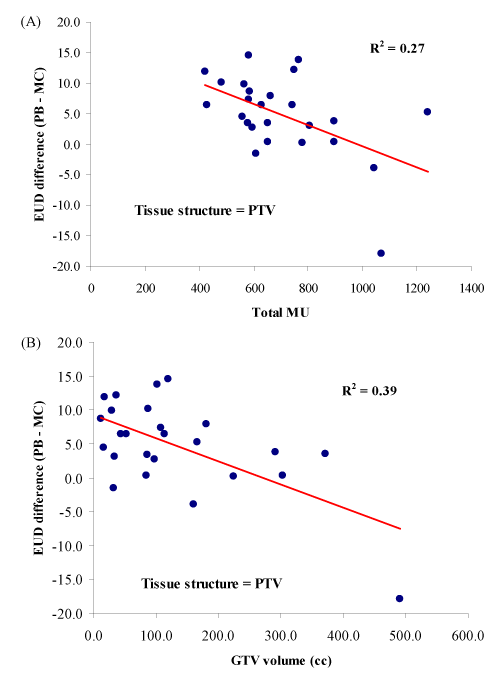Figure 3: Plot of PTV EUD difference (PB – MC) between the two algorithms versus (a) Total MU with regression coeffi cients β = -0.017 EUD/MU, p = 0.01, and (b) GTV volume (cc) with regression coeffi cients β = -0.034 EUD/cc, p < 0.01.